Custom SearchInterpolation It is frequently necessary to estimate the value of an angle to a closer approximation than is available in the table. This is equivalent to estimating between table entries, and the process is called INTERPOLATION. For example in the foregoing problem it was determined that the angle value was between 22 36 and 22 42. The following paragraphs describe the procedure for interpolating to find a closer approximation to the value of the angle. The following arrangement of numbers is recommended for interpolation: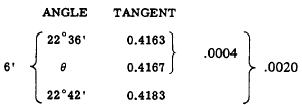The spread between 22 36 and 22 42 is 6, and we use the comparison of the tangent values to determine how much of this 6 spread is included in q , the angle whose value is sought. Notice that the tangent of q is different from tan 22 36 by only 0.0004, and the total spread in the tangent values is 0.0020. Therefore, the tangent of q is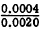- of the way between the tangents of the two angles given in the table. This is 1/5 of the total spread, since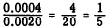Another way of arriving at this result is to observe that the total spread is 20 ten-thousandths, and that the partial spread corresponding to angle q is 4 ten-thousandths. Since 4 out of 20 is the same as 1 out of 5, angle q is l/5 of the way between 22 36 and 22 42. Taking 1/5 of the 6 spread between the angles, we have the following calculation: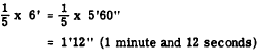The 12" obtained in this calculation causes our answer to appear to have greater accuracy than the tables from which it is derived. This apparent increase in accuracy is a normal result of interpolation. Final answers based on interpolated data should be rounded off to the same degree of accuracy as that of the original data. The value of 1 minute and 12 seconds found in the foregoing problem is added to 22 36, as follows: q = 22 36 + 1'12" = 223712" Therefore q is 22 37, approximately. The foregoing problem could have been solved in terms of tenths and hundredths of a degree, rather than minutes, as follows:In this example, we are concerned with an angular spread of 0.10 and q is located 1/5 of the way through this spread. Thus we have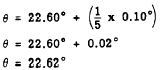Interpolation must be approached with common sense, in order to avoid applying corrections in the wrong direction. For example, the cosine of an angle decreases in value as the angle increases from 0 to 90. If we need the value of the cosine of an angle such as 2239, the calculation is as follows: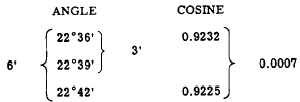In this example, it is easy to see that 2239 is halfway between 2236 and 2242. Therefore the cosine of 2239 is halfway between the cosine of 2236 and that of 2242. Taking one-half of the spread between these cosines, we then SUBTRACT from 0.9232 to find the cosine of 2239, as follows:Practice problems: 1. Use the table in appendix II to find the decimal value of each of the following ratios:2. Find the angle which corresponds to each of the following decimal values in appendix II: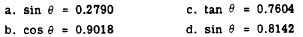Answers:Integrated Publishing, Inc. - A (SDVOSB) Service Disabled Veteran Owned Small Business